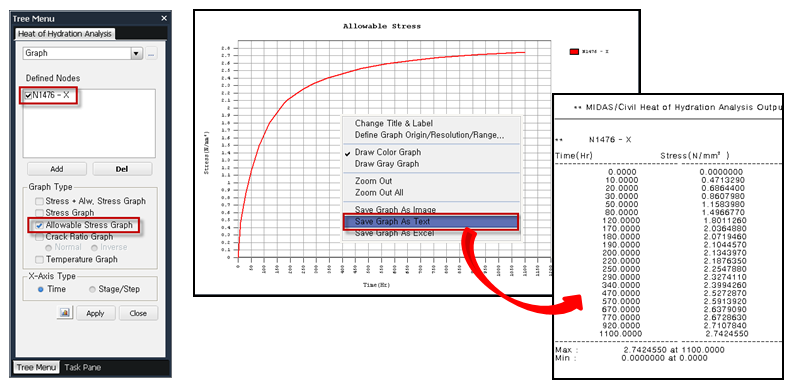Civil Created Edited

# How can we check the tensile strength value in hydration heat analysis?

## Question

How can we check the tensile strength value in hydration heat analysis?

The tensile strength can be checked in 'Results tab > Results group > HY Results > Graph' after the analysis is completed.

The main purpose of hydration heat analysis is to review the cracks occurring in concrete and provide countermeasures for crack control. The thermal cracking index is calculated by the following equation:The tensile strength is automatically defined based on the compressive strength and specified variables entered in 'Properties tab > Time Dependent Material group > Compressive Strength'.The tensile strength defined as described above can be checked in the 'Results tab > Results group > HY Results > Graph' after analysis.

(According to the JCI 2008 standards, the tensile strength can also be checked by selecting 'Tensile Strength' in the graph of the Compressive Strength dialog box. However, the tensile strength obtained here is not the effective tensile strength, but the tensile strength for the normal strength.)

The Allowable Stress Graph in the following figure represents the tensile strength graph, and by clicking the Apply button, you can check the result values of the selected item in the graph.0